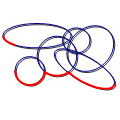CGAL 5.3 - 2D Envelopes
2D Envelopes ReferenceRon Wein
This package consits of functions that computes the lower (or upper) envelope of a set of arbitrary curves in 2D. The output is represented as an envelope diagram, namely a subdivision of the $$x$$-axis into intervals, such that the identity of the curves that induce the envelope on each interval is unique.
Introduced in: CGAL 3.3
Depends on: 2D Arrangements
BibTeX: cgal:w-e2-21b

This package consists of functions that compute the lower (or upper) envelope of a set of arbitrary curves in 2D. The output is represented as an envelope diagram, namely a subdivision of the $$x$$-axis into intervals, such that the identity of the curves that induce the envelope over each interval is unique.

## Functions

• CGAL::lower_envelope_2
• CGAL::upper_envelope_2
• CGAL::lower_envelope_x_monotone_2
• CGAL::upper_envelope_x_monotone_2

## Concepts

• EnvelopeDiagram_1
• EnvelopeDiagramVertex
• EnvelopeDiagramEdge

## Classes

• CGAL::Envelope_diagram_1<Traits>

Concepts

## Classes

class  CGAL::Envelope_diagram_1< Traits >
This class is the default envelope-diagram class used by envelope functions to represent the minimization or the maximization diagram of a set of curves. More...

## Functions

template<class InputIterator , class EnvelopeDiagram >
void CGAL::lower_envelope_2 (InputIterator begin, InputIterator end, EnvelopeDiagram &diag)
Computes the lower envelope of a set of curves in $$\mathbb{R}^2$$, as given by the range [begin, end). More...

template<class InputIterator , class EnvelopeDiagram >
void CGAL::lower_envelope_x_monotone_2 (InputIterator begin, InputIterator end, EnvelopeDiagram &diag)
Computes the lower envelope of a set of $$x$$-monotone curves in $$\mathbb{R}^2$$, as given by the range [begin, end). More...

template<class InputIterator , class EnvelopeDiagram , class Traits >
void CGAL::lower_envelope_x_monotone_2 (InputIterator begin, InputIterator end, EnvelopeDiagram &diag, const Traits &traits)
Computes the lower envelope of a set of $$x$$-monotone curves in $$\mathbb{R}^2$$, as given by the range [begin, end) with the help of the arrangement traits object traits responsible for their creation. More...

template<class InputIterator , class EnvelopeDiagram >
void CGAL::upper_envelope_2 (InputIterator begin, InputIterator end, EnvelopeDiagram &diag)
Computes the upper envelope of a set of curves in $$\mathbb{R}^2$$, as given by the range [begin, end). More...

template<class InputIterator , class EnvelopeDiagram >
void CGAL::upper_envelope_x_monotone_2 (InputIterator begin, InputIterator end, EnvelopeDiagram &diag)
Computes the upper envelope of a set of $$x$$-monotone curves in $$\mathbb{R}^2$$, as given by the range [begin, end). More...

template<class InputIterator , class EnvelopeDiagram , class Traits >
void CGAL::upper_envelope_x_monotone_2 (InputIterator begin, InputIterator end, EnvelopeDiagram &diag, const Traits &traits)
Computes the upper envelope of a set of $$x$$-monotone curves in $$\mathbb{R}^2$$, as given by the range [begin, end) with the help of the arrangement traits object traits responsbile for their creation. More...

## ◆ lower_envelope_2()

template<class InputIterator , class EnvelopeDiagram >
 void CGAL::lower_envelope_2 ( InputIterator begin, InputIterator end, EnvelopeDiagram & diag )

#include <CGAL/envelope_2.h>

Computes the lower envelope of a set of curves in $$\mathbb{R}^2$$, as given by the range [begin, end).

The lower envelope is represented using the output minimization diagram diag.

Template Parameters
 InputIterator must be an input iterator with value type EnvelopeDiagram::Traits_2::Curve_2. EnvelopeDiagram must be a model of the concept EnvelopeDiagram_1.
Examples:
Envelope_2/envelope_circles.cpp.

## ◆ lower_envelope_x_monotone_2() [1/2]

template<class InputIterator , class EnvelopeDiagram >
 void CGAL::lower_envelope_x_monotone_2 ( InputIterator begin, InputIterator end, EnvelopeDiagram & diag )

#include <CGAL/envelope_2.h>

Computes the lower envelope of a set of $$x$$-monotone curves in $$\mathbb{R}^2$$, as given by the range [begin, end).

The lower envelope is represented using the output minimization diagram diag.

Template Parameters
 InputIterator must be an input iterator with value type EnvelopeDiagram::X_monotone_curve_2. EnvelopeDiagram must be a model of the concept EnvelopeDiagram_1.
Examples:
Envelope_2/convex_hull_2.cpp, and Envelope_2/envelope_segments.cpp.

## ◆ lower_envelope_x_monotone_2() [2/2]

template<class InputIterator , class EnvelopeDiagram , class Traits >
 void CGAL::lower_envelope_x_monotone_2 ( InputIterator begin, InputIterator end, EnvelopeDiagram & diag, const Traits & traits )

#include <CGAL/envelope_2.h>

Computes the lower envelope of a set of $$x$$-monotone curves in $$\mathbb{R}^2$$, as given by the range [begin, end) with the help of the arrangement traits object traits responsible for their creation.

Reusing the same traits object improves speed if the traits class caches data. The lower envelope is represented using the output minimization diagram diag.

Template Parameters
 InputIterator must be an input iterator with value type EnvelopeDiagram::X_monotone_curve_2. EnvelopeDiagram must be a model of the concept EnvelopeDiagram_1. Traits must be a model of the concept ArrangementXMonotoneTraits_2.

## ◆ upper_envelope_2()

template<class InputIterator , class EnvelopeDiagram >
 void CGAL::upper_envelope_2 ( InputIterator begin, InputIterator end, EnvelopeDiagram & diag )

#include <CGAL/envelope_2.h>

Computes the upper envelope of a set of curves in $$\mathbb{R}^2$$, as given by the range [begin, end).

The upper envelope is represented using the output maximization diagram diag.

Template Parameters
 InputIterator must be an input iterator with value type EnvelopeDiagram::Traits_2::Curve_2. EnvelopeDiagram must be a model of the concept EnvelopeDiagram_1.
Examples:
Envelope_2/envelope_circles.cpp.

## ◆ upper_envelope_x_monotone_2() [1/2]

template<class InputIterator , class EnvelopeDiagram >
 void CGAL::upper_envelope_x_monotone_2 ( InputIterator begin, InputIterator end, EnvelopeDiagram & diag )

#include <CGAL/envelope_2.h>

Computes the upper envelope of a set of $$x$$-monotone curves in $$\mathbb{R}^2$$, as given by the range [begin, end).

The upper envelope is represented using the output maximization diagram diag.

Template Parameters
 InputIterator must be an input iterator with value type EnvelopeDiagram::X_monotone_curve_2. EnvelopeDiagram must be a model of the concept EnvelopeDiagram_1.
Examples:
Envelope_2/convex_hull_2.cpp.

## ◆ upper_envelope_x_monotone_2() [2/2]

template<class InputIterator , class EnvelopeDiagram , class Traits >
 void CGAL::upper_envelope_x_monotone_2 ( InputIterator begin, InputIterator end, EnvelopeDiagram & diag, const Traits & traits )

#include <CGAL/envelope_2.h>

Computes the upper envelope of a set of $$x$$-monotone curves in $$\mathbb{R}^2$$, as given by the range [begin, end) with the help of the arrangement traits object traits responsbile for their creation.

Reusing the same traits object improves speed if the traits class caches data. The upper envelope is represented using the output maximization diagram diag.

Template Parameters
 InputIterator must be an input iterator with value type EnvelopeDiagram::X_monotone_curve_2. EnvelopeDiagram must be a model of the concept EnvelopeDiagram_1. Traits must be a model of the concept ArrangementXMonotoneTraits_2.